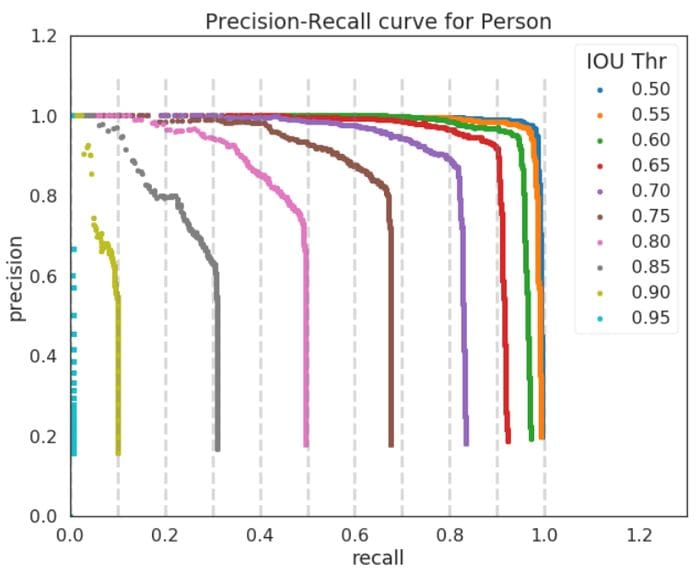# Metrics to Use to Evaluate Deep Learning Object Detectors

Different approaches have been employed to solve the growing need for accurate object detection models. More recently, with the popularization of the convolutional neural networks (CNN) and GPU-accelerated deep-learning frameworks, object- detection algorithms started being developed from a new perspective. CNNs such as R-CNN, Fast R-CNN, Faster R-CNN, R-FCN, SSD and Yolo have highly increased the performance standards on the field.

Once you have trained your first object detector, the next step is to know its performance. Sure enough, you can see the model finds all the objects in the pictures you feed it. Great! But how do you quantify that? How should we decide which model is better?

Since the classification task only evaluates the probability of the class object appearing in the image, it is a straightforward task for a classifier to identify correct predictions from incorrect ones. However, the object detection task localizes the object further with a bounding box associated with its corresponding confidence score to report how certain the bounding box of the object class is detected.

A detector outcome is commonly composed of a list of bounding boxes, confidence levels and classes, as seen in the following Figure:Object detection metrics serve as a measure to assess how well the model performs on an object detection task. It also enables us to compare multiple detection systems objectively or compare them to a benchmark. In most competitions, the average precision (AP) and its derivations are the metrics adopted to assess the detections and thus rank the teams.

### Understanding the various metric:

IoU:
Guiding principle in all state-of-the-art metrics is the so-called Intersection-over-Union (IoU) overlap measure. It is quite literally defined as the intersection over union of the detection bounding box and the ground truth bounding box.

Dividing the area of overlap between predicted bounding box and ground truth by the area of their union yields the Intersection over Union.

An Intersection over Union score > 0.5 is normally considered a “good” prediction.IoU metric determines how many objects were detected correctly and how many false positives were generated (will be discussed below).

True Positives [TP]

Number of detections with IoU>0.5

False Positives [FP]

Number of detections with IoU<=0.5 or detected more than once

False Negatives [FN]

Number of objects that not detected or detected with IoU<=0.5

Precision

Precision measures how accurate your predictions are. i.e. the percentage of your predictions that are correct.

Precision = True positive / (True positive + False positive)

Recall

Recall measures how good you find all the positives.

Recall = True positive / (True positive + False negative)

F1 Score

F1 score is HM (Harmonic Mean) of precision and recall.AP

The general definition for the Average Precision(AP) is finding the area under the precision-recall curve.

mAP

The mAP for object detection is the average of the AP calculated for all the classes. mAP@0.5 means that it is the mAP calculated at IOU threshold 0.5.mAP Vs other metric

The mAP is a good measure of the sensitivity of the neural network. So good mAP indicates a model that’s stable and consistent across different confidence thresholds. Precision, Recall and F1 score are computed for given confidence threshold.

If ‘model A’ has better Precision, Recall and F1 score than ‘model B’ but say mAP of ‘model B’ is better than ‘model B’,  scenario indicates that either ‘model B’ has very bad recall at higher confidence thresholds or very bad precision at lower confidence thresholds. So higher Precision, Recall and F1 score of ‘model A’ indicate that at that confidence threshold it’s better in terms of all the 3 metrics compared to that of ‘model B’.### Which metric is more important ?

In general to analyse better performing models, it’s advisable to use both validation set (data set that is used to tune hyper-parameters) and test set (data set that is used to assess the performance of a fully-trained model).

On validation set

• Use mAP to select the best performing model (model that is more stable and consistent) out of all the trained weights across iterations/epochs. Use mAP to understand whether the model should be trained/tuned further or not.
• Check class level AP values to ensure the model is stable and good across the classes.
• As per use-case/application, if you’re completely tolerant to FNs and highly intolerant to FPs then to train/tune the model accordingly use Precision.
• As per use-case/application, if you’re completely tolerant to FPs and highly intolerant to FNs then to train/tune the model accordingly use Recall.

On test set

• If you’re neutral towards FPs and FNs, then use F1 score to evaluate the best performing model.
• If FPs are not acceptable to you (without caring much about FNs) then pick the model with higher Precision
• If FNs are not acceptable to you (without caring much about FPs) then pick the model with higher Recall
• Once you decide metric you should be using, try out multiple confidence thresholds (say for example – 0.25, 0.35 and 0.5) for given model to understand for which confidence threshold value the metric you selected works in your favour and also to understand acceptable trade off ranges (say you want Precision of at least 80% and some decent Recall). Once confidence threshold is decided, you use it across different models to find out the best performing model.

References

Bio: Venkatesh Wadawadagi is a solution consultant at Sahaj Software Solutions. He helps businesses solve complex problems using AI-powered solutions. He specialises in Deep Learning, Computer Vision, Machine Learning, NLP(Natural Language Processing), embedded-AI, business intelligence and data analytics.

Related: